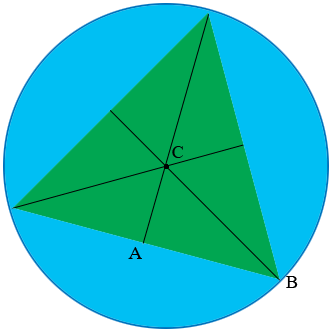SEARCH HOMEMath Central Quandaries & QueriesQuestion from Sumit: what is the area (in sq. unit) of an equilateral triangle inscribed in the circle x^2+y^2-4x-6y-23=0.Hi,

Do you know how to find the radius of the circle from the equation?

In my diagram $C$ is the center of the circle that has an equilateral triangle inscribed.What do you know about the triangle $ABC?$

Write back if you need more assistance,
PennyMath Central is supported by the University of Regina and The Pacific Institute for the Mathematical Sciences.